# Fundamentals of Engineering Economics

## Quiz 10 : Project Cash-Flow AnalysisStudy FlashcardsLooking for Economics Homework Help?

## Quiz 10 :Project Cash-Flow Analysis

Question TypeScottsdale Print Shop expects to have an annual taxable income of $300,000 from its regular business over the next two years. The company is also considering the proposed acquisition of a new printing machine to expand current business to offer in its product catalog. The machine's installed price is$105,000. The machine falls into the MACRS five-year class, and it will have an estimated salvage value of $30,000 at the end of six years. The machine is expected to generate additional before-tax revenue of$120,000 per year. (a) Determine the company's annual marginal (incremental) tax rates over the next two years with the proposed investment in the printing machine. (b) Determine the company's annual average tax rates over the next two years with the machine.
Free
Essay

Given information:
• A print shop's taxable income is worth of $300,000. • Price of new machine is$105,000.
• New machine depreciates in 5 years MACRS rate.• Salvage value at the end of 6 th year is $30,000. • Before tax annual revenue is$120,000.
a.Marginal tax rate:
Table-1 shows the marginal tax rate.
Table-1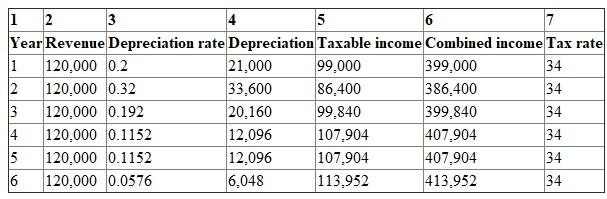Steps:
• Before tax annual revenue for 6 years is presented in Column 2.
• 5 years MACRS rate depreciation rate is presented in Column 3.
• Depreciation of new machine is calculated in Column 4, where new machine price is multiplied with Column 3.
• Taxable income is calculated in Column 5 (Column 2 minus Column 4).
• Combined income is calculated in Column 6, which is obtained by adding taxable income after project (in Column 5) and taxable income after project ($300,000). • Marginal tax rate is shown in Column 7. Since the income in 6 years range between$10 million to $15 million, marginal tax rate is 34 percent. b.Average tax: Average tax rate is the percentage of taxable amount on total income.Table-2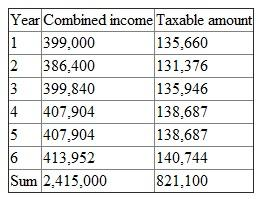Hence, the average tax rate is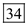percent. Tags Choose question tagA new absorption chiller system costs$360,000 and will save $52,500 in each of the next 12 years. The asset is classified as a seven-year MACRS property for depreciation purpose. The expected salvage value is$20,000. The firm pays taxes at a combined rate of 40% and has an MARR of 12%. What is the net present worth of the system? (a) $46,725 (b)$63,739 (c) $62,112 (d)$53,317
Free
Multiple Choice

Given information:
• Cost of new system is $360,000. • Savings per year is$52,500.
• Salvage value is 20,000.
• Combined tax rate is 40 percent.
• Minimum annual rate of return is 12 percent.
• Time period is 12 years.
Recovery rate for 7 year:
Table-1 shows the recovery rate under the General Depreciation System for 7 year
Table-1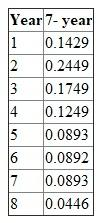First year depreciation:
Depreciation can be calculated by using the following formula.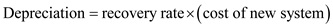…… (1)Substitute the respective values in Equation (1) to calculate the depreciation in first year.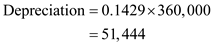Hence, the depreciation in the first year is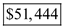.
Depreciation for all the years:
Hence, similar calculation is done in Table-2 to obtain the depreciation by using the Equation (1).
Table-2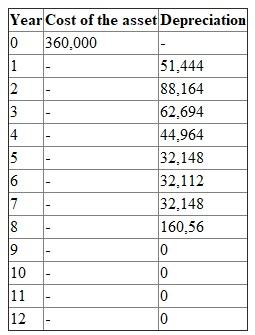First year net cash flow:
Net cash flow can be calculated by using the following formula.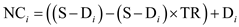…… (2)Where,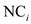= net cash flow of the i th year
S = savings per year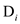= depreciation of the i th year
TR = tax rate
Substitute the respective values in Equation (2) to calculate the first year net cash flow.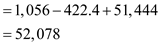Hence, the first year net cash flow is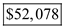.
Taxable gain:
Taxable gain can be calculated by using the following formula. When the asset is disposed, half year depreciation should be calculated.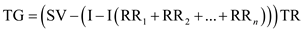…… (3)Where,
TG = taxable gain
TR = tax rate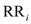=recovery rate of i th year
SV = salvage value
Substitute the respective values in Equation (3) to calculate the taxable gain.Taxable gain is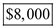.
Net cash flow for all the year:
Hence, similar calculation is done in Table-3 to obtain the net cash flow by using the Equation (2) Final year net cash flow can be obtained by adding the salvage value and subtracting the capital gain.
Table -3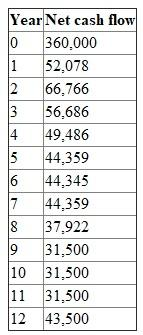In 12 th year cash flow is sum of annual cash flow worth of $31,500 and salvage value worth of$20,000 minus taxable gain worth of $8,000. Present value: Present value of net cash flow can be calculated by using the following formula: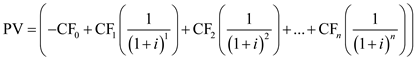…… (4)Where, i = interest n = time period PV = present value SV = salvage value CG = capital gain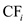= cash flow of i th year Substitute the respective values in Equation (4) to calculate the present value of the net cash flow.The present value of the cash flow is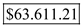. Hence, option b is correct. Tags Choose question tagSuppose ADI Corporation's break-even sales volume is$500,000 with fixed costs of $250,000. (a) Compute the contribution margin percentage. (b) Compute the selling price if variable costs are$15 per unit.
Free
Essay

Given information:
• Sales volume of a company is $500,000. • Fixed cost is$250,000.
a.Contribution margin percentage:
Contribution margin percentage can be obtained by using the following formula: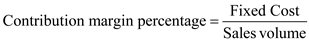…… (1)Substitute the respective values in Equation (1) to obtain the contribution margin percentage.Hence, the contribution margin percentage is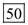percentage.
b.Per unit selling price:
The contribution margin percentage is equal to 0.5 and variable cost is $15 per unit; hence, the selling price is as follows:Hence, the per unit selling price is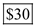. Tags Choose question tagUsing the replacement chain method (machine B can be replaced with an identical machine at the end of year 3), determine which project should be adopted after tax. (a) Machine A. (b) Machine B. (c) Either machine. (d) Neither machine. Multiple Choice Answer: Tags Choose question tagGiven the following information, answer the questions: The ratio of variable cost per unit divided by selling price per unit equals 0.3. Fixed costs amount to$60,000. (a) Draw the cost-volume-profit diagram. (b) What is the break-even point? (c) What effect would an 8% decrease in selling price have on the break-even point from part (b)?
Essay
Tags
Choose question tagSuppose that in Problem 10s.2, the firm borrowed the entire capital investment at 10% interest over 10 years. If the required principal and interest payments in year 10 are Principal payment: $14,795 Interest payment:$1,480, What would be the net cash flow at the end of year 10? (a) $46,725 (b)$63,000 (c) $62,112 (d)$53,317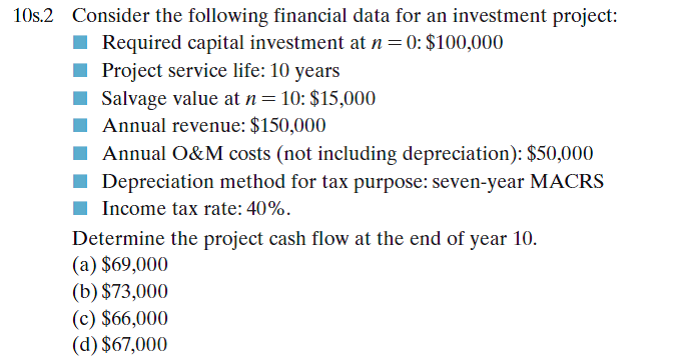Multiple Choice
Tags
Choose question tagConsider the following financial data for an investment project: Required capital investment at n = 0: $100,000 Project service life: 10 years Salvage value at n = 10:$15,000 Annual revenue: $150,000 Annual O M costs (not including depreciation):$50,000 Depreciation method for tax purpose: seven-year MACRS Income tax rate: 40%. Determine the project cash flow at the end of year 10. (a) $69,000 (b)$73,000 (c) $66,000 (d)$67,000
Multiple Choice
Tags
Choose question tagWhat is the internal rate of return (after tax) of machine A? (a) 28% (b) 39% (c) 35% (d) 43%
Multiple Choice
Tags
Choose question tagA firm is trying to choose between two machines to manufacture a new line of office furniture. The financial data for each machine have been compiled as follows: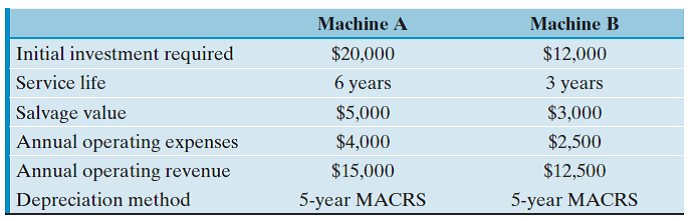The firm's marginal tax rate is 40% and uses a 15% discount rate to value the projects. Also, assume that the required service period is indefinite.
Essay
Tags
Choose question tagThe Austin Electric Company has three product lines of surge protectors commonly used in PC and other electronic devices-A, B, and C-having a contribution margin of $3,$2, and $1, respectively. The president foresees sales of 200,000 units in the coming period, consisting of 20,000 units of A, 100,000 units of B, and 80,000 units of C. The company's fixed costs for the period are$255,000. (a) What is the company's break-even point in units, assuming that the given sales mix is maintained? (b) If the mix is maintained, what is the total contribution margin when 200,000 units are sold? What is operating income? (c) What would be operating income if 20,000 units of A, 80,000 units of B, and 100,000 units of C were sold? What is the new break-even point in units if these relationships persist in the next period?
Essay
Tags
Choose question tagIdentify which of the following costs are fixed and which are variable: (a) Wages paid to temporary workers (b) Property taxes on a factory building (c) Property taxes on an administrative building (d) Sales commission (e) Electricity for machinery and equipment in a plant (f) Heat and air conditioning for a plant (g) Salaries paid to design engineers (h) Regular maintenance on machinery and equipment (i) Basic raw materials used in production (j) Factory fire insurance
Essay
Tags
Choose question tagWhat is the net present worth of machine B after tax over 3 years? (a) $6,394 (b)$6,233 (c) $5,562 (d)$7,070
Multiple Choice
Tags
Choose question tagBuffalo Environmental Service expects to generate a taxable income of $350,000 from its regular business in 2012. The company is also considering a new venture: cleaning up oil spills made by fishing boats in lakes. This new venture is expected to generate an additional taxable income of$180,000. (a) Determine the firm's marginal tax rates before and after the venture. (b) Determine the firm's average tax rates before and after the venture.
Essay
Tags
Choose question tagSuppose that a company expects the following financial results from a project during its first year operation: Sales revenue: $300,000 Variable costs:$100,000 Fixed costs: $50,000 Total unit produced and sold: 10,000 units (a) Compute the contribution margin percentage. (b) Compute the break-even point in units sold. Essay Answer: Tags Choose question tagClassify the following costs into either being product cost or period cost: (a) Raw material costs (b) Income taxes paid (c) Interest expenses on borrowed funds (d) Wages incurred in producing products (e) Fire insurance premium paid on factory buildings (f) Electric bill for the warehouse operation (g) Salary paid for engineers (h) Material handling cost related to production (i) Salary paid for plant manager (j) Leasing expense for forklift trucks in warehouse operation (k) Mortgage payments on factory buildings Essay Answer: Tags Choose question tagA corporation is considering purchasing a machine that costs$120,000 and will save $X per year after taxes. The cost of operating the machine, including maintenance and depreciation, is$20,000 per year after taxes. The machine will be needed for four years after which it will have a zero salvage value. If the firm wants a 14% rate of return after taxes, what is the minimum after-tax annual savings that must be generated to realize a 14% rate of return after taxes? (a) $50,000 (b)$61,184 (c) $91,974 (d)$101,974
Multiple Choice
Tags
Choose question tagSome commonly known costs associated with manufacturing operations are listed below: (a) Paint shop superintendent's salary (b) Labor costs in assembling a product (c) Rent on a factory building (d) Radio-frequency identification (RFID) units embedded in the final product during shipping (e) Depreciation on machinery (f) Lubricants used for machines (g) CPU chips used in notebook production (h) Paint used in automobile production (i) Janitorial and custodial salaries (j) Coffee beans used in packaging roasted coffee (k) Sugar used in icecream production (l) Electricity for operation of machines (m) Electricity for heating and cooling the factory building (n) Glue used in electronic board production Classify each cost as being either variable or fixed with respect to volume or level of activity.
Essay
Tags
Choose question tagA corporation is considering purchasing a machine that will save $200,000 per year before taxes. The cost of operating the machine, including maintenance, is$80,000 per year. The machine costing $150,000 will be needed for five years, after which it will have a salvage value of$25,000. A straight-line depreciation with no half-year convention applies (i.e., 20% each year). If the firm wants 15% rate of return after taxes, what is the net present value of the cash flows generated from this machine? The firm's income tax rate is 40%. (a) $137,306 (b)$218,313 (c) $199,460 (d)$375,000
Multiple ChoiceThe accompanying figures depict a number of cost behavior patterns that might be found in a company's cost structure. The vertical axis on each graph represents total cost, and the horizontal axis on each graph represents level of activity (volume). For each of the given situations, identify the graph that illustrates the cost pattern involved. Any graph may be used more than once. (Adapted originally from the CPA exam; also found in R. H. Garrison and E. W. Noreen, Managerial Accounting, 9th edition, Irwin, 2009.) (a) Electricity bill-a flat-rate fixed charge plus a variable cost after a certain number of kilowatt-hours are used. (b) City water bill, which is computed as follows: First 1,000,000 gallons $1,000 flat, or less Next 10,000 gallons$0.003 per gallon used Next 10,000 gallons $0.006 per gallon used Next 10,000 gallons$0.009 per gallon used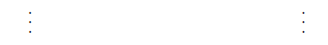(c) Depreciation of equipment, where the amount is computed by the straight-line method. When the depreciation rate was established, it was anticipated that the obsolescence factor would be greater than the wear-and-tear factor. (d) Rent on a factory building donated by the city, where the agreement calls for a fixed-fee payment unless 200,000 labor-hours or more are worked, in which case no rent need be paid. (e) Cost of raw materials, where the cost decreases by 5 cents per unit for each of the first 100 units purchased after which it remains constant at $2.50 per unit. (f) Salaries of maintenance workers, where one maintenance worker is needed for every 1,000 machine hours or less (that is, 0 to 1,000 hours require one maintenance worker, 1,001 to 2,000 hours require two maintenance workers, etc.). (g) Cost of raw materials used. (h) Rent on a factory building donated by the county, where the agreement calls for rent of$100,000 less $1 for each direct labor-hour worked in excess of 200,000 hours, but a minimum rental payment of$20,000 must be paid. (i) Use of a machine under a lease, where a minimum charge of $1,000 is paid for up to 400 hours of machine time. After 400 hours of machine time, an additional charge of$2 per hour is paid up to a maximum charge of $2,000 per period.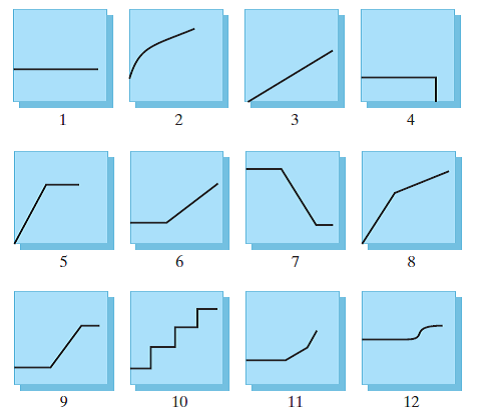Essay Answer: Tags Choose question tagJ J Electric Company expects to have taxable income of$320,000 from its regular business over the next two years. The company is considering a new residential wiring project for a proposed apartment complex during year 0. This two-year project requires purchase of new equipment for $30,000. The equipment falls into the MACRS five-year class. The equipment will be sold after two years for$12,000. The project will bring in additional revenue of $100,000 each year, but it is expected to incur an additional operating cost of$40,000 each year. What is the income tax rate to use in year 1 for this project evaluation? (a) 39% (b) 34% (c) 33.77% (d) 35.39%